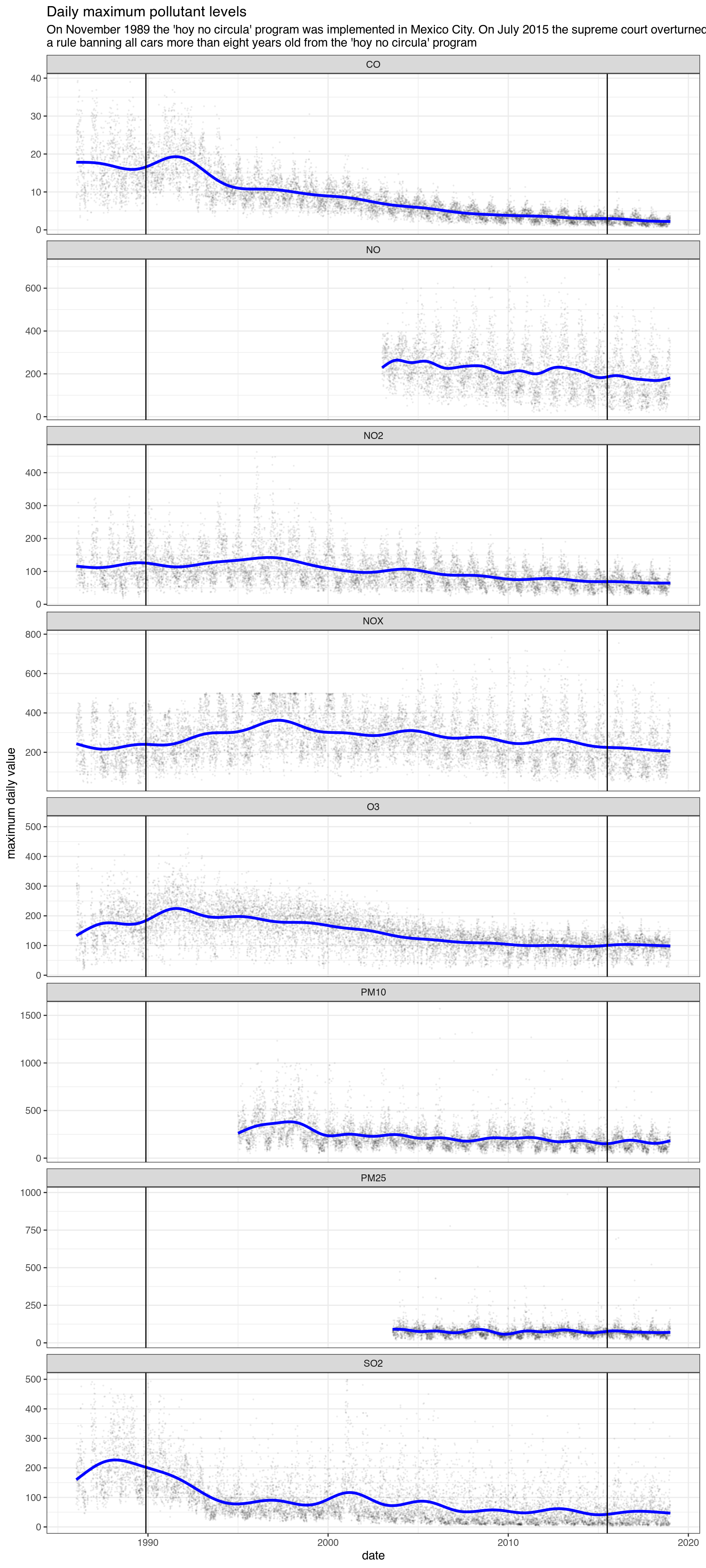In an effort to lower pollution levels the Mexico City government introduced in 1989 the Hoy no circula program, a ban on most cars from circulating once a week. But did it really work?

## Station data

The function get_station_data can be used to download pollution and wind data going back to 1986, although not all pollutants are available that far back, and requesting a pollutant that is not available will result in a warning.

## Auto-install required R packages
if (!require("pacman")) install.packages("pacman")
## Loading required package: pacman
pacman::p_load(aire.zmvm, dplyr, ggplot2, mgcv, lubridate, stringr,
gridExtra, zoo, sp, mapproj)
download_data <- function(pollutant) {
suppressWarnings({
o3 <- get_station_data(criterion = "MAXIMOS", # Can be MAXIMOS (daily maximum),
# MINIMOS (daily minimum),
# or HORARIOS (hourly average)
pollutant = pollutant, # "SO2", "CO", "NOX", "NO2", "NO", "O3",
# "PM10", "PM25", "WSP", "WDR", "TMP", "RH"
year = 1986:2018, # The earliest year allowed is 1986
progress = FALSE)
})
o3\$pollutant <- pollutant
# Daily max among all base stations
o3 %>%
group_by(date, pollutant) %>%
summarise(max = ifelse(all(is.na(value)),
NA,
base::max(value, na.rm = TRUE))) %>%
na.omit() %>%
ungroup()
}

pollutant = c("NO", "SO2", "CO", "NOX", "NO2", "O3", "PM10", "PM25"),
SIMPLIFY = FALSE)
df_maximos <- bind_rows(ll)
knitr::kable(head(df_maximos))
date pollutant max
2003-01-01 NO 201
2003-01-02 NO 228
2003-01-03 NO 229
2003-01-04 NO 301
2003-01-05 NO 230
2003-01-06 NO 275

We can plot all pollutants going back to 1986 and add a trend line based on a GAM controlling for month of year, with lines for the start of the hoy no circula and the supreme court decision that the hoy no circula doesn’t necessarily apply to older cars. A more complex model is left as an exercise to the reader.

fitted <- function(max, month, date, year) {
df=data.frame(max = max,
month = month,
date = date,
year = year)
fit <- gam(max ~ s(month, bs = "cc", k = 12) + s(as.numeric(date), k = 20),
data = df, correlation = corARMA(form = ~ 1|year, p = 1))
predict(fit, newdata = df, type = "terms")[,2] + mean(max)
}
print("test")
##  "test"
df_maximos <- df_maximos %>%
mutate(month = month(date),
year = year(date))%>%
group_by(pollutant) %>%
mutate(pred = fitted(max,month,date,year)) %>%
ungroup()
print("test2")
##  "test2"
# Plot the daily highest level with trendline
ggplot(df_maximos,
aes(date, max, group = 1)) +
geom_point(color = "black", size = .2, alpha = .05) +
geom_line(aes(date, pred), color ="blue", size = 1.2)  +
labs(title = "Daily maximum pollutant levels",
subtitle = "On November 1989 the 'hoy no circula' program was implemented in Mexico City. On July 2015 the supreme court overturned\na rule banning all cars more than eight years old from the 'hoy no circula' program") +
ylab("maximum daily value") +
xlab("date") +
geom_vline(xintercept = as.numeric(as.Date("1989-11-20"))) +
geom_vline(xintercept = as.numeric(as.Date("2015-07-01"))) +
theme_bw() +
facet_wrap(~pollutant, scales = "free_y", ncol = 1)